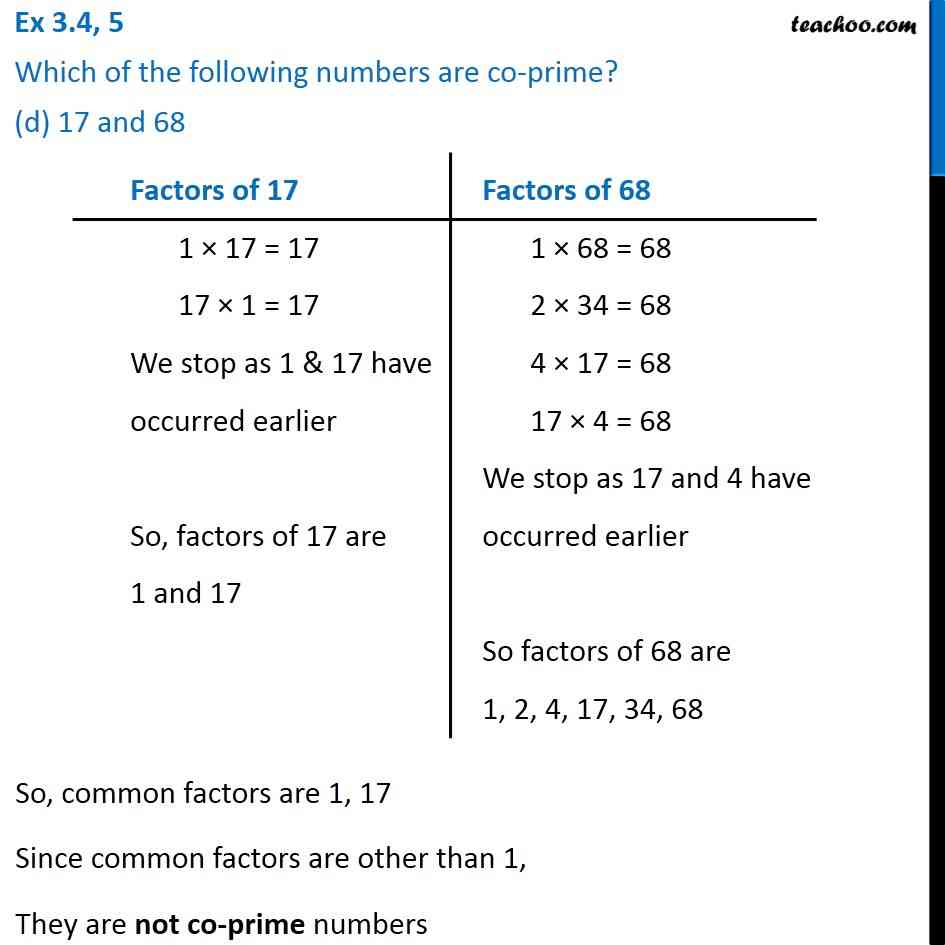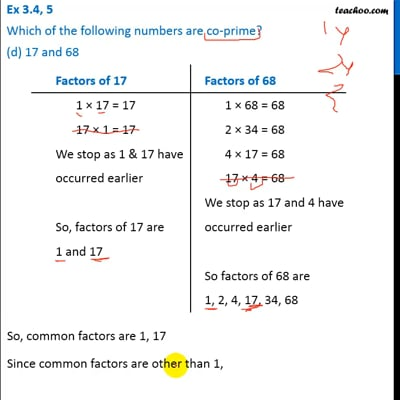Ex 3.4

Chapter 3 Class 6 Playing with Numbers
Serial order wiseThis video is only available for Teachoo black users

Learn in your speed, with individual attention - Teachoo Maths 1-on-1 Class

### Transcript

Ex 3.4, 5 Which of the following numbers are co-prime? (d) 17 and 68 Factors of 17 1 × 17 = 17 17 × 1 = 17 We stop as 1 & 17 have occurred earlier So, factors of 17 are 1 and 17 Factors of 68 1 × 68 = 68 2 × 34 = 68 4 × 17 = 68 17 × 4 = 68 We stop as 17 and 4 have occurred earlier So factors of 68 are 1, 2, 4, 17, 34, 68 So, common factors are 1, 17 Since common factors are other than 1, They are not co-prime numbers